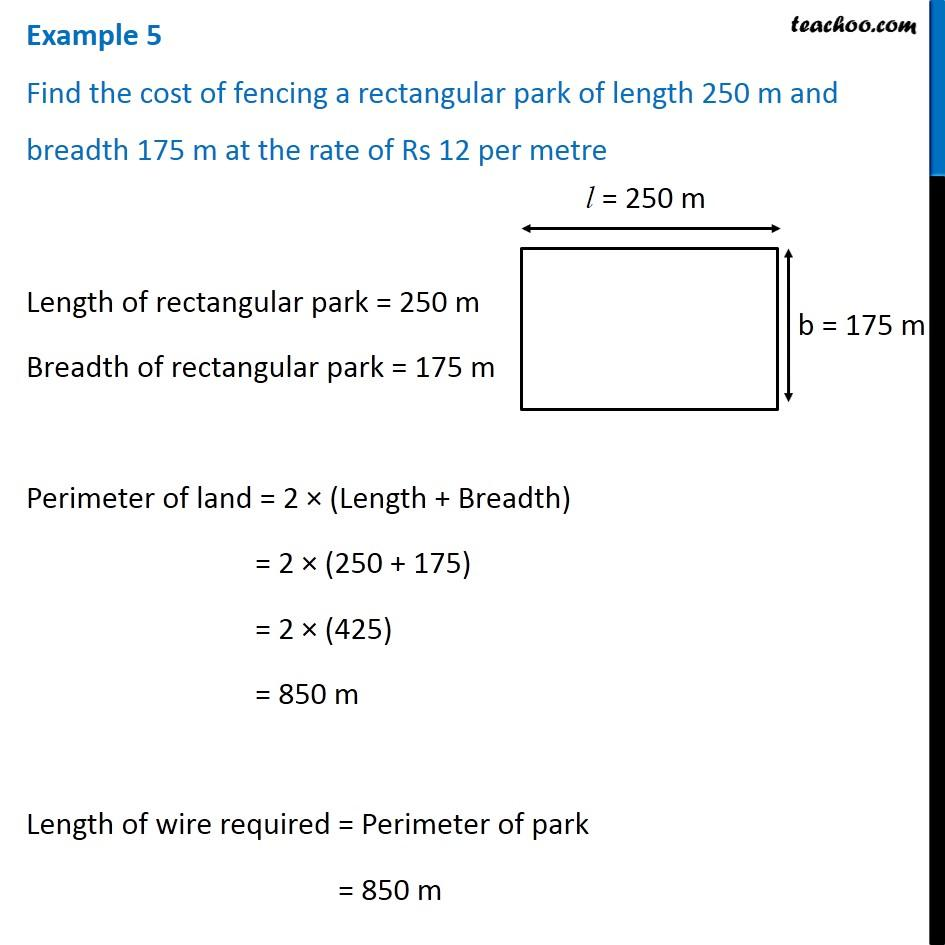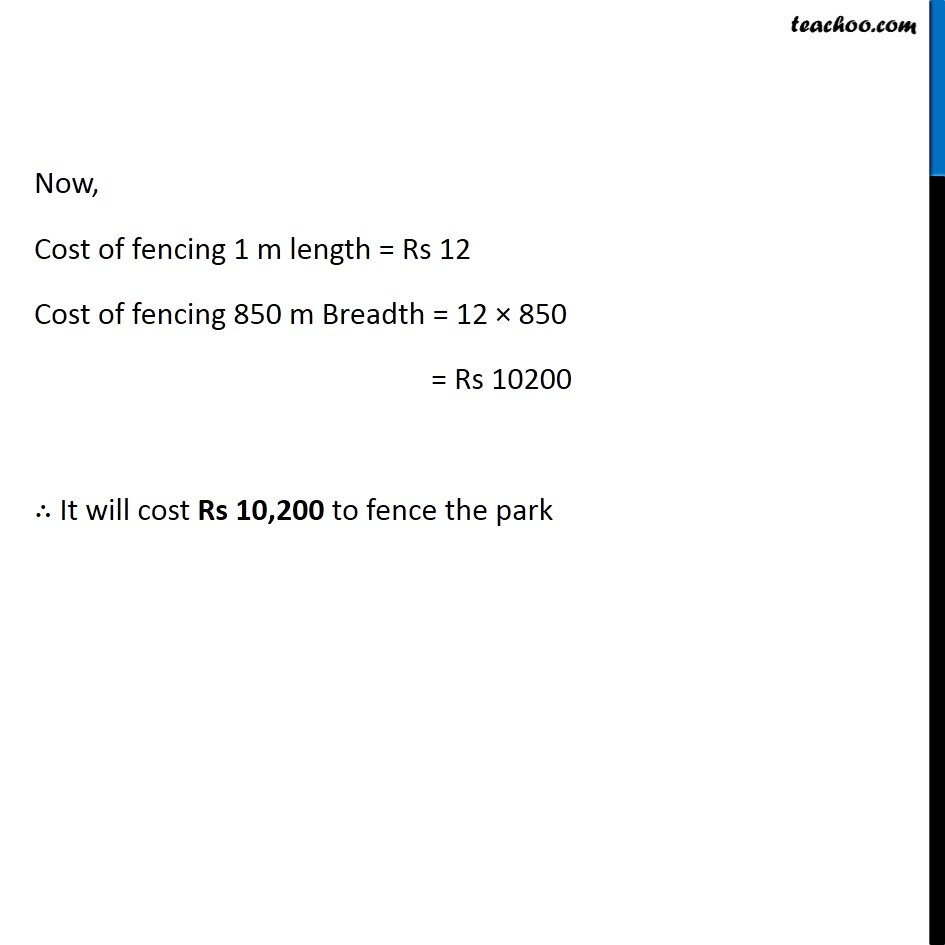Perimeter of Rectangle

Chapter 10 Class 6 Mensuration
Concept wiseGet live Maths 1-on-1 Classs - Class 6 to 12

### Transcript

Example 5 Find the cost of fencing a rectangular park of length 250 m and breadth 175 m at the rate of Rs 12 per metre Length of rectangular park = 250 m Breadth of rectangular park = 175 m Perimeter of land = 2 × (Length + Breadth) = 2 × (250 + 175) = 2 × (425) = 850 m Length of wire required = Perimeter of park = 850 m Now, Cost of fencing 1 m length = Rs 12 Cost of fencing 850 m Breadth = 12 × 850 = Rs 10200 ∴ It will cost Rs 10,200 to fence the park